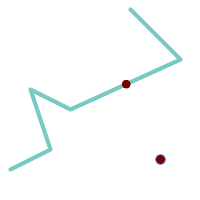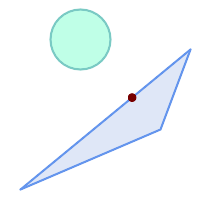## Name

ST_ClosestPoint — Returns the 2D point on g1 that is closest to g2. This is the first point of the shortest line from one geometry to the other.

## Synopsis

`geometry ST_ClosestPoint(`geometry geom1, geometry geom2`)`;

`geography ST_ClosestPoint(`geography geom1, geography geom2, boolean use_spheroid = true`)`;

## Description

Returns the 2-dimensional point on `geom1` that is closest to `geom2`. This is the first point of the shortest line between the geometries (as computed by ST_ShortestLine).If you have a 3D Geometry, you may prefer to use ST_3DClosestPoint.

Enhanced: 3.4.0 - Support for geography.

Availability: 1.5.0

## ExamplesThe closest point for a Point and a LineString is the point itself. The closest point for a LineString and a Point is a point on the line.

```SELECT ST_AsText( ST_ClosestPoint(pt,line)) AS cp_pt_line,
ST_AsText( ST_ClosestPoint(line,pt)) AS cp_line_pt
FROM (SELECT 'POINT (160 40)'::geometry AS pt,
'LINESTRING (10 30, 50 50, 30 110, 70 90, 180 140, 130 190)'::geometry AS line ) AS t;

cp_pt_line   |                cp_line_pt
----------------+------------------------------------------
POINT(160 40)  | POINT(125.75342465753425 115.34246575342466)
```The closest point on polygon A to polygon B

```SELECT ST_AsText( ST_ClosestPoint(
'POLYGON ((190 150, 20 10, 160 70, 190 150))',
ST_Buffer('POINT(80 160)', 30)	)) As ptwkt;
------------------------------------------
POINT(131.59149149528952 101.89887534906197)
```